So far we always used RGB colors in our shaders, meaning the components of our color vector always map to the red green and blue components of the color. This is great if we want to render the color or tint it, but adjusting the hue or saturation becomes very bothersome. For those kinds of operations we can use the HSV color space. In addition to the HSV color space there are also other similar color spaces, like the HSL or CIE color models. Some of them are very similar to the HSL model while others get way closer to the visible spectrum at the cost of higher cost of calculating them. For simplicities sake I’m only going to explain the HSV model here.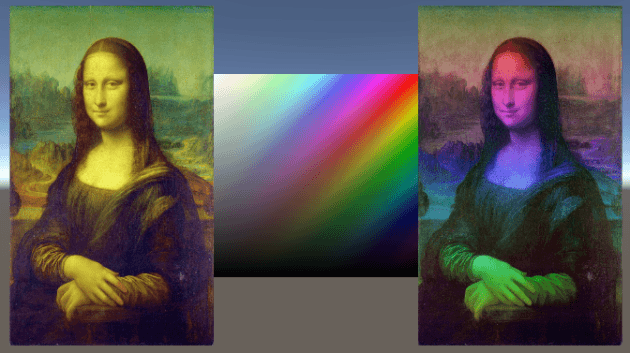## How does it work?

When using the HSV model we also have 3 components which define our color, but in this case they map to the hue, saturation and value of the color. Because the maximum and minimum value of the hue map to the same value (red), we can view it as a circle. This concept can be taken a step further to imagine the color space as a cylinder where the hue is the rotation around the center, the saturation is the proximity to the center and the value is represented by the relative height of the point in the cylinder.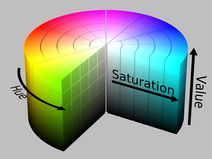## Generating a RGB Color from Hue

The most critical step in converting colors from HSV to RGB is to convert the hue of a HSV color to a RGB color, that’s why we’re writing a function to do only this. In our implementation the hue will be between 0 and 1. Other implementations define it to be between 0 and 360, similar to degree numbers in a circle, but I personally prefer 0 to 1 scaling since it makes it easier to work with functions like `saturate` or `frac` which assume we’re working in those dimensions.

In the range from 0 to 1 each of the 3 components has one third where it has a value of 1, one third where it has a value of 0 and two sixths where it’s linearly growing from 0 to 1 or decreasing from 1 to 0 accordingly. Those changes in values are offset in a way that each hue generates a different color.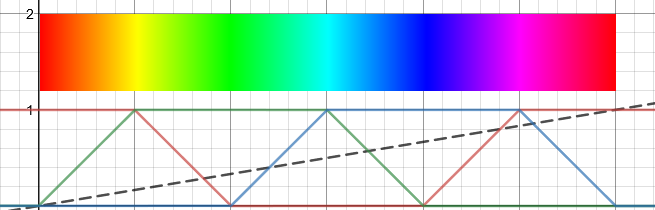In code we can most efficiently represent this by taking the absolute value of a value that’s first multiplied by 6(because it has to reach a value of 1 over the change of a sixth) and shifted to the side. The green and blue values both go up and then down again in the range, that’s why they are subtracted from 2, flipping them. The red value instead first decreases and then later increases again. To archieve this, 1 is subtracted from it.

After the increase and decrease of the values is set up the values are combined and the saturate function is called on it. The saturate function ensures that no value is below 0 or above 1.

If we want to make sure that hue values above 1 or below 0 don’t result in a red hue and instead wrap around the color spectrum like expected we can just take the fractional part of the hue and ignore the decimal part. In hlsl, the `frac` function does exactly that.

``````float3 hue2rgb(float hue) {
hue = frac(hue); //only use fractional part of hue, making it loop
float r = abs(hue * 6 - 3) - 1; //red
float g = 2 - abs(hue * 6 - 2); //green
float b = 2 - abs(hue * 6 - 4); //blue
float3 rgb = float3(r,g,b); //combine components
rgb = saturate(rgb); //clamp between 0 and 1
return rgb;
}
``````

After setting up this method you can simply use it in any other method to generate a rgb color with a specific hue.

``````//the fragment shader
fixed4 frag(v2f i) : SV_TARGET{
float3 col = hue2rgb(i.uv);
return float4(col, 1);
}
``````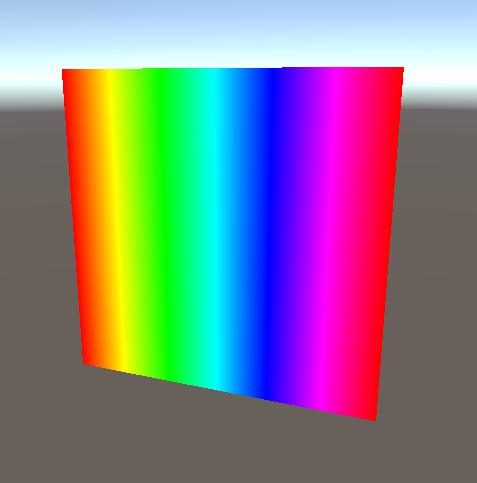## Full HSV to RGB conversion

After being able to convert the hue into a rgb color that looks correct we next also have to make the output color respect the saturation and value. To apply the saturation to the already generated color, we do a linear interpolation from 1 to the color and use the saturation component of the vector as the argument. Since 1 stands for full white in thic context, this makes the hue vanish for low saturation color while preserving it for high saturation ones.

The last step to take is to appy the value. Since the value stands for the brightness of the color the operation to apply it is to simply multiply the color so far by the value component.

``````float3 hsv2rgb(float3 hsv)
{
float3 rgb = hue2rgb(hsv.x); //apply hue
rgb = lerp(1, rgb, hsv.y); //apply saturation
rgb = rgb * hsv.z; //apply value
return rgb;
}
``````

To test this we can make a new example shader. In this one I used the x uv coordinate as the saturation, the y coordinate as the value and generated the hue by taking a value that increases diagonally by subtracting the y from the x UV component.

``````//the fragment shader
fixed4 frag(v2f i) : SV_TARGET
{
float diagonal = i.uv.x - i.uv.y;
float3 col = hsv2rgb(float3(diagonal, i.uv.x, i.uv.y));
return float4(col, 1);
}
``````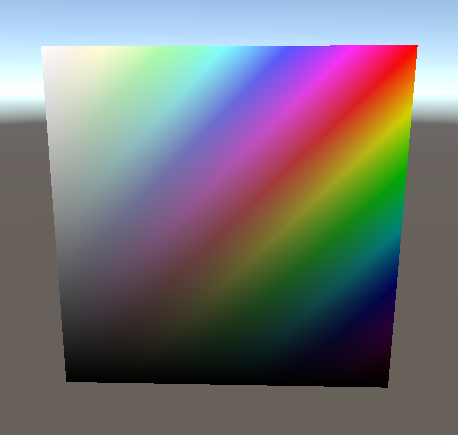## RGB to HSV conversion

Unlike the conversion from rgb to hsv, the data we’re using to generate the hsv color is a bit more entangled between the different components of the output vector so we won’t split this into several functions.

Which variables we’re using to get the hue depends on which component of the rgb color has the highest value, additionally we also need the difference between the highest and lowest component to calculate it. So after calculating the highest and lowest components of the input color via the builtin `min` and `max` functions and using them to get the difference between them we first create the hue and then check which of the components is equal to the highest value. We then subtract the two values that are not the highest value from each other, divide them by the difference between minimum and maximum value and then add 0, 2 or 4 depending on the color that’s the highest. Afterwards we divide the resulting hue by 6 and only use the fractional part.

By getting the biggest component we ensure that the other 2 components are the minimum component and the component that’s changing in the third we’re in right now (see graph further up the article). For example when red is the most intense color, either blue has the lowest value and the difference between green to blue is calculated or green has the lowest value, in that case the resulting difference has a negative value. One thing that distorts this value is that because the value and saturation are also part of the input value, the hue might be way off from the “completely red/green/blue” points, but since max and min values are super close the difference we just calculated is still very small. This is luckily easy to fix by dividing the difference by the difference between the biggest and smallest component of the input color we calculated earlier. With those modifications we get a value of 0 if the colors that aren’t the biggest color are the same, a.k.a. the hue is red/green/blue or a value of -1/1 if it’s yellow/magenta/cyan and a value inbetween for the other hues. By adding a value based on the hue of the most intense input component we’re remapping the colors to -1 to 1 for the redish colors, 1 to 3 for the greenish colors and 3 to 5 for the blueish colors. The division afterwards pulls this into the range of -1/6 to 5/6 and taking the fractional part of that makes the negative values wrap around so it’s in the range of 0 to 1 as expected.

Getting the saturation and value is easier. The saturation is the difference between the biggest and smallest component, divided by the biggest component. The division factors out the multiplication by the value we do in the hsv to rgb conversion. To get the value we can just take the biggest component of the input value, since neither applying the hue nor the saturation can make the highest value drop below 1, so everything that goes into it is dependent on the value of the color.

``````float3 rgb2hsv(float3 rgb)
{
float maxComponent = max(rgb.r, max(rgb.g, rgb.b));
float minComponent = min(rgb.r, min(rgb.g, rgb.b));
float diff = maxComponent - minComponent;
float hue = 0;
if(maxComponent == rgb.r) {
hue = 0+(rgb.g-rgb.b)/diff;
} else if(maxComponent == rgb.g) {
hue = 2+(rgb.b-rgb.r)/diff;
} else if(maxComponent == rgb.b) {
hue = 4+(rgb.r-rgb.g)/diff;
}
hue = frac(hue / 6);
float saturation = diff / maxComponent;
float value = maxComponent;
return float3(hue, saturation, value);
}
``````

With this done, you can now convert a color into hsv, adjust it and move it back into rgb to render the color. The easiest one is to add some value to the hue to make it shift in a rainbow effect.

``````//the fragment shader
fixed4 frag(v2f i) : SV_TARGET{
float3 col = tex2D(_MainTex, i.uv);
float3 hsv = rgb2hsv(col);
hsv.x += i.uv.y + _Time.y * _CycleSpeed;
col = hsv2rgb(hsv);
return float4(col, 1);
}
``````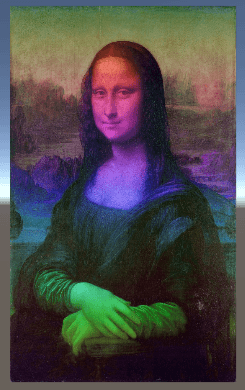While with the hue you can just add values where a change of 1 results in the same hue again, 0.5 is the opposite hue etc, the saturation and value should usually be kept between 0 and 1. To adjust them we can use power operator. Taking the `N`th power of the saturation or value where `N` is above 1 makes the color less saturated/darker. Taking the `N`th power with `N` between 0 and 1 makes the color more saturated/brighter. With this knowledge we can make a shader that adjusts those properties in the shader. It’s important to keep in mind that you shouldn’t do that just to statically adjust a image though, since the conversions as well as taking the power of a number are pretty expensive operations, instead consider to change the image in a image manipulation program or if you want to use shaders, via shadron or the texture baking tool I wrote a tutorial on.

The fragment function of a shader adjusting all components of the HSV color could look like this.

``````//the fragment shader
fixed4 frag(v2f i) : SV_TARGET{
float3 col = tex2D(_MainTex, i.uv);
float3 hsv = rgb2hsv(col);
hsv.x = hsv.x + _HueShift;
hsv.y = pow(hsv.y, _SaturationPower);
hsv.z = pow(hsv.z, _ValuePower);
col = hsv2rgb(hsv);
return float4(col, 1);
}
``````

## Source

I used include files in the building of those examples, I explain how to use them more extensively in the tutorial about random number generation.

### Function Library

``````#ifndef HSV_LIB
#define HSV_LIB

float3 hue2rgb(float hue) {
hue = frac(hue); //only use fractional part
float r = abs(hue * 6 - 3) - 1; //red
float g = 2 - abs(hue * 6 - 2); //green
float b = 2 - abs(hue * 6 - 4); //blue
float3 rgb = float3(r,g,b); //combine components
rgb = saturate(rgb); //clamp between 0 and 1
return rgb;
}

float3 hsv2rgb(float3 hsv)
{
float3 rgb = hue2rgb(hsv.x); //apply hue
rgb = lerp(1, rgb, hsv.y); //apply saturation
rgb = rgb * hsv.z; //apply value
return rgb;
}

float3 rgb2hsv(float3 rgb)
{
float maxComponent = max(rgb.r, max(rgb.g, rgb.b));
float minComponent = min(rgb.r, min(rgb.g, rgb.b));
float diff = maxComponent - minComponent;
float hue = 0;
if(maxComponent == rgb.r) {
hue = 0+(rgb.g-rgb.b)/diff;
} else if(maxComponent == rgb.g) {
hue = 2+(rgb.b-rgb.r)/diff;
} else if(maxComponent == rgb.b) {
hue = 4+(rgb.r-rgb.g)/diff;
}
hue = frac(hue / 6);
float saturation = diff / maxComponent;
float value = maxComponent;
return float3(hue, saturation, value);
}

#endif
``````

### HSV to RGB Test

``````Shader "Tutorial/041_HSV/HueTest"{
//the material is completely non-transparent and is rendered at the same time as the other opaque geometry
Tags{ "RenderType"="Opaque" "Queue"="Geometry"}

Pass{
CGPROGRAM

#include "UnityCG.cginc"
#include "HSVLibrary.cginc"

#pragma vertex vert
#pragma fragment frag

//the object data that's put into the vertex shader
struct appdata{
float4 vertex : POSITION;
float2 uv : TEXCOORD0;
};

//the data that's used to generate fragments and can be read by the fragment shader
struct v2f{
float4 position : SV_POSITION;
float2 uv : TEXCOORD0;
};

v2f vert(appdata v){
v2f o;
//convert the vertex positions from object space to clip space so they can be rendered
o.position = UnityObjectToClipPos(v.vertex);
o.uv = v.uv;
return o;
}

fixed4 frag(v2f i) : SV_TARGET{
float diagonal = i.uv.x - i.uv.y;
float3 col = hsv2rgb(float3(diagonal, i.uv.x, i.uv.y));
return float4(col, 1);
}

ENDCG
}
}
}
``````

### Hue Cycle

``````Shader "Tutorial/041_HSV/HueCycle"{
//show values to edit in inspector
Properties{
_CycleSpeed ("Hue Cycle Speed", Range(0, 1)) = 0
_MainTex ("Texture", 2D) = "white" {}
}

//the material is completely non-transparent and is rendered at the same time as the other opaque geometry
Tags{ "RenderType"="Opaque" "Queue"="Geometry"}

Pass{
CGPROGRAM

#include "UnityCG.cginc"
#include "HSVLibrary.cginc"

#pragma vertex vert
#pragma fragment frag

//Hue cycle speed
float _CycleSpeed;

sampler2D _MainTex;
float4 _MainTex_ST;

//the object data that's put into the vertex shader
struct appdata{
float4 vertex : POSITION;
float2 uv : TEXCOORD0;
};

//the data that's used to generate fragments and can be read by the fragment shader
struct v2f{
float4 position : SV_POSITION;
float2 uv : TEXCOORD0;
};

v2f vert(appdata v){
v2f o;
//convert the vertex positions from object space to clip space so they can be rendered
o.position = UnityObjectToClipPos(v.vertex);
o.uv = v.uv;
return o;
}

fixed4 frag(v2f i) : SV_TARGET{
float3 col = tex2D(_MainTex, i.uv);
float3 hsv = rgb2hsv(col);
hsv.x += i.uv.y + _Time.y * _CycleSpeed;
col = hsv2rgb(hsv);
return float4(col, 1);
}

ENDCG
}
}
}
``````

``````Shader "Tutorial/041_HSV/Adjust"{
//show values to edit in inspector
Properties{
_HueShift("Hue Shift", Range(-1, 1)) = 0
[PowerSlider(10.0)]_SaturationPower("Saturation Adjustment", Range(10.0, 0.1)) = 1
[PowerSlider(10.0)]_ValuePower("Value Adjustment", Range(10.0, 0.1)) = 1
_MainTex ("Texture", 2D) = "white" {}
}

//the material is completely non-transparent and is rendered at the same time as the other opaque geometry
Tags{ "RenderType"="Opaque" "Queue"="Geometry"}

Pass{
CGPROGRAM

#include "UnityCG.cginc"
#include "HSVLibrary.cginc"

#pragma vertex vert
#pragma fragment frag

//HSV modification variables
float _HueShift;
float _SaturationPower;
float _ValuePower;

sampler2D _MainTex;
float4 _MainTex_ST;

//the object data that's put into the vertex shader
struct appdata{
float4 vertex : POSITION;
float2 uv : TEXCOORD0;
};

//the data that's used to generate fragments and can be read by the fragment shader
struct v2f{
float4 position : SV_POSITION;
float2 uv : TEXCOORD0;
};

v2f vert(appdata v){
v2f o;
//convert the vertex positions from object space to clip space so they can be rendered
o.position = UnityObjectToClipPos(v.vertex);
o.uv = v.uv;
return o;
}

fixed4 frag(v2f i) : SV_TARGET{
float3 col = tex2D(_MainTex, i.uv);
float3 hsv = rgb2hsv(col);
hsv.x = hsv.x + _HueShift;
hsv.y = pow(hsv.y, _SaturationPower);
hsv.z = pow(hsv.z, _ValuePower);
col = hsv2rgb(hsv);
return float4(col, 1);
}

ENDCG
}
}
}
``````

As always thank you so much for reading and supporting me, your messages of support mean the world to me 💖.

You can also find me on twitter at @totallyRonja. If you liked my tutorial and want to support me you can do that on Patreon (patreon.com/RonjaTutorials) or Ko-Fi (ko-fi.com/RonjaTutorials).